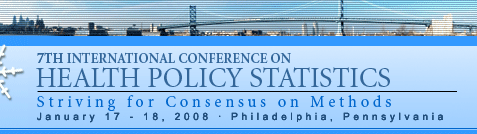Robust regression approach to the analysis of cost data Giulia Barbati, Department of Public Health and Microbiology, University of Turin, Italy  Dario Gregori, Department of Public Health and Microbiology, University of Turin, Italy  Eva Pagano, Unit of Cancer Epidemiology, University of Turin, CERMS and CPO-Piemonte, Italy  *Michele Petrinco, Unit of Cancer Epidemiology, University of Turin, Italy  Keywords: cost; cost analysis; robust regression Recent research in parametric robust estimation proposes estimators for regression models with asymmetric error distribution:the ‘Truncated Maximum Likelihood’ estimators. We compared results obtained by TML with GLM Gamma model. An initial high breakdown point but inefficient S-estimate is computed;observations that are unlikely under the estimated model are rejected;the maximum likelihood estimate is computed with the retained observations. In a simulation study,the TML showed a satisfactory performance for large sample sizes,comparable to the Gamma.In presence of simulated outliers,ATML estimator performs better in terms of coverage and MSE the greater the level of outliers.On real data,coefficients and their significance differed across models showing sensitivity to outliers. We estimated a mean value less sensitive to outliers and the covariates effects with smaller std error.

Print Friendly